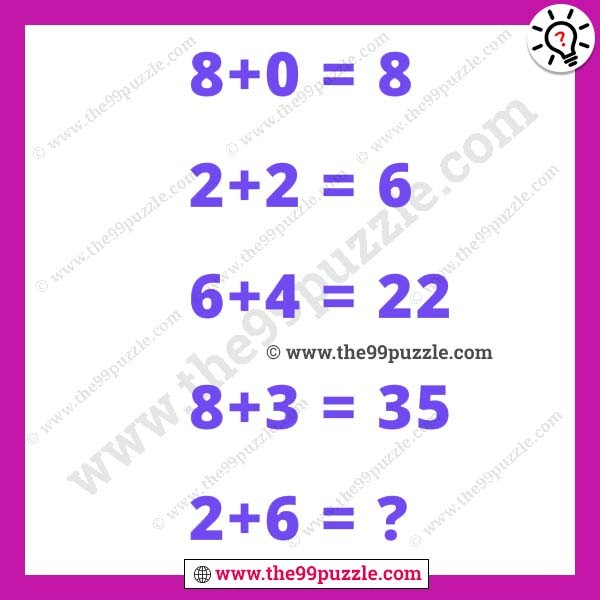# Quick math brain teaser with answer – Puzz241

Only genius solves this quick math brain teaser. The mathematical puzzle is one type of brain game. This reasoning puzzle can crack your mind. Puzzle lovers like to solve various types of brain teasers puzzles. Practice more puzzles that you make more genius and smarter. All mathematical puzzle questions have math tricks. You have to crack the math tricks and easily solve the puzzles. In this math puzzle, you see some tricky math equations. If you are a genius in mathematics you can quickly solve this puzzle.

8+0 = 8

2+2 = 6

6+4 = 22

8+3 = 35

2+6 = ?###### Explanation:

8+0 = (8×1+0) = 8

2+2 = (2×2+2) = 6

6+4 = (6×3+4) = 22

8+3 = (8×4+3) = 35

2+6 = (2×5+6) = 16